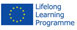This project has been funded with support from the European Commission.
This material reflects the views only of the author, and the Commission cannot be held responsible for any use which may be made of the information contained therein

Also available in: bg es it lv pl ro sk en fb# Experiences

Homepage > Experiences > Teachers Experiences

TEACHERS EXPERIENCES FORM

Title of the Experience
Lined plane. Verifying the rule of the parallelogram.
Name of the teacher
Gomboli Sandro
Country where it took place
Italy
School typology
Vocational School
Thematic Area
Physics
Experience typology
Laboratory
Type of contact
Direct
Description of the Experience
he understanding of the concepts of material point and rigid body constraint and degrees of freedom of a body are fundamental to solving problems of static.
The study of the conditions of equilibrium of a body on an inclined plane, allows a better understanding of the force exerted by the constraint and the parallelogram rule applied to a concrete example.
The experience consists of the direct measurement with dynamometer of the force needed to maintain a balance between a metal cylinder on an inclined plane
The measures of the force are carried out in five different configurations by setting as many heights of the inclined plane.
It simplifies the study of the conditions of equilibrium of the cylinder approximating a value of a material point
The first equation of static allows us to determine the vectorial relationship between the three forces that act on the cylinder or better on its center of gravity, force of gravity, constraining force, and elastic force. Through the rule of the parallelogram the force of gravity is decomposed because it is measured directly by a dynamometer in two forces, a parallel and perpendicular to the inclined plane.
The system of three forces thus turns into a similar system of four forces with the advantage of being able to simplify the vectorial equation into a system of two algebraic equations
Through geometric considerations on the proportionality of the sides of two similar triangles it calculates the value of the parallel component of the force of gravity.
It then compares this indirect measure of the force with its direct measurement carried out with a dynamometer tested in five configurations their approximate equality within the limits of experimental approximate values, thus demonstrating the validity of the rule of the parallelogram.
The study of the inclined plane one of the most well-known simple tools, implies an understanding of the nature of the constraints and forces constraining and presupposes the knowledge of the rule of the parallelogram in the sum of vector quantities when they have directions that do not concord.
These are the latest findings and on them we will focus particular attention, recovering and deepening the knowledge and the potential for the analysis of the propagation of approximate values in indirect measures especially in the calculation of the constraining forces and their approximate values.
By plotting a graph we will check the direct proportionality between the height of the inclined plane, and the elastic force that maintains equilibrium in the inclined plane a metal cylinder.
For more able classes in calculation you can indirectly measure the constraining force of the inclined plane in the various configurations by applying the Pythagorean theorem.
The difficulty of this measure is in the determination of the approximate value propagated, but this difficulty can be overcome with appropriate mathematical considerations which can be very useful because they allow students to better understand the rules of the propagation of approximate values and calculation methods quite frequently in the processing of experimental data.
To have a further confirmation of the validity of the rule of the parallelogram you can also derive the value of the balancing force by the use of graphics.
Choosing a suitable scale you draw on a sheet of graph paper the force of gravity and the elastic force with the right direction, direction and intensity and obtain the parallelogram that will allow us by measuring with a ruler the segment perpendicular to the inclined plan to obtain the intensity of the third force, that of the constraint.